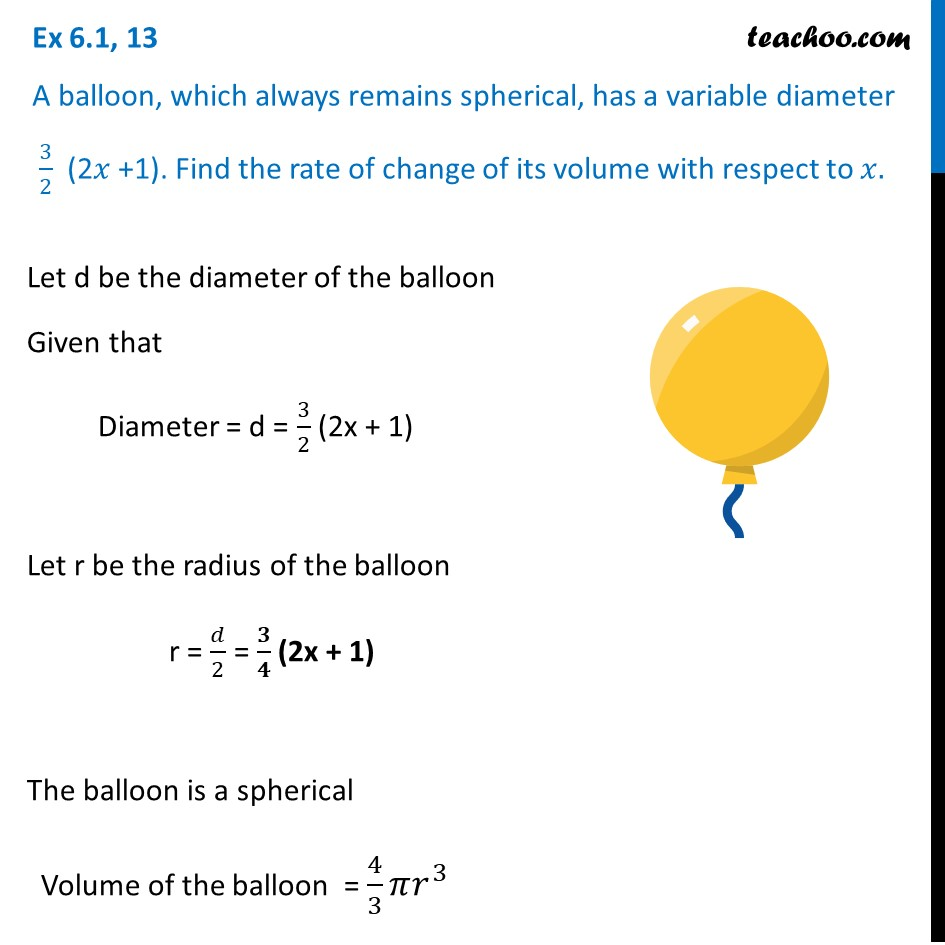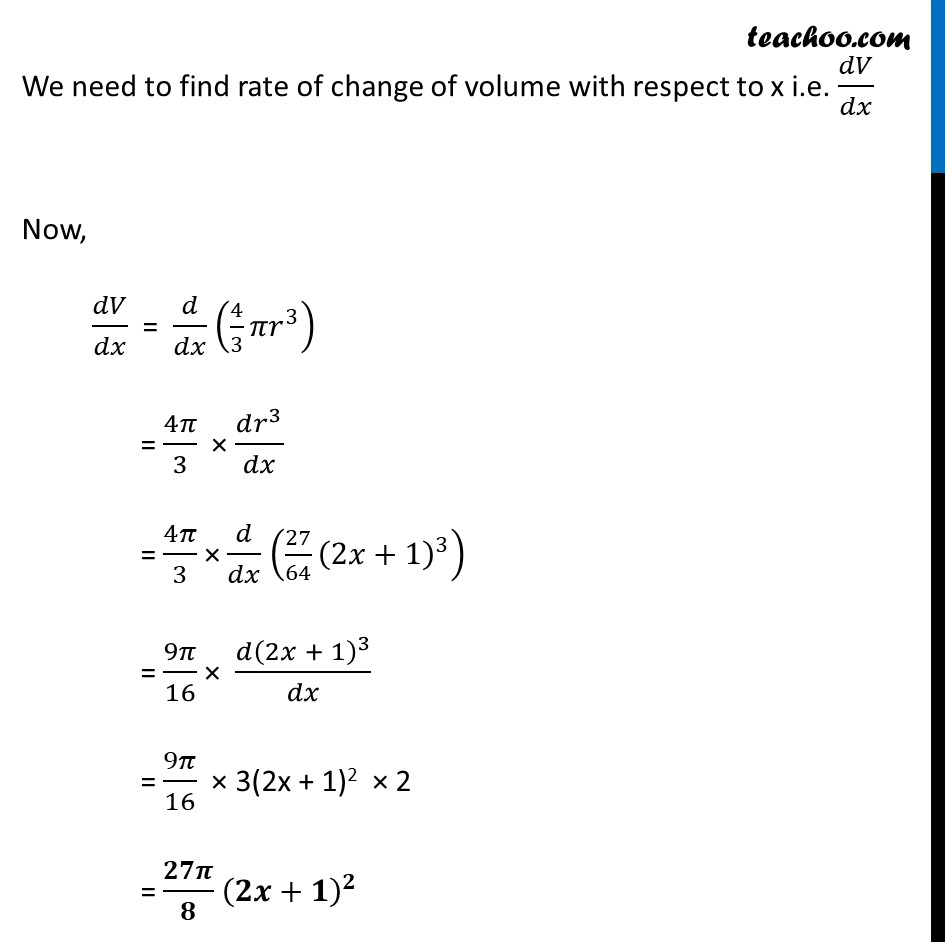Finding rate of change

Chapter 6 Class 12 Application of Derivatives
Concept wiseLearn in your speed, with individual attention - Teachoo Maths 1-on-1 Class

### Transcript

Ex 6.1, 13 A balloon, which always remains spherical, has a variable diameter 3/2 (2𝑥 +1). Find the rate of change of its volume with respect to 𝑥.Let d be the diameter of the balloon Given that Diameter = d = 3/2 (2x + 1) Let r be the radius of the balloon r = 𝑑/2 = 𝟑/𝟒 (2x + 1) The balloon is a spherical Volume of the balloon = 4/3 𝜋𝑟^3 We need to find rate of change of volume with respect to x i.e. 𝑑𝑉/𝑑𝑥 Now, 𝑑𝑉/𝑑𝑥 = 𝑑/𝑑𝑥 (4/3 𝜋𝑟^3 ) = 4𝜋/3 × (𝑑𝑟^3)/𝑑𝑥 = 4𝜋/3 × 𝑑/𝑑𝑥 (27/64 (2𝑥+1)^3 ) = 9𝜋/16 × (𝑑(2𝑥 + 1)^3)/𝑑𝑥 = 9𝜋/16 × 3(2x + 1)2 × 2 = 𝟐𝟕𝝅/𝟖 (𝟐𝒙+𝟏)^𝟐